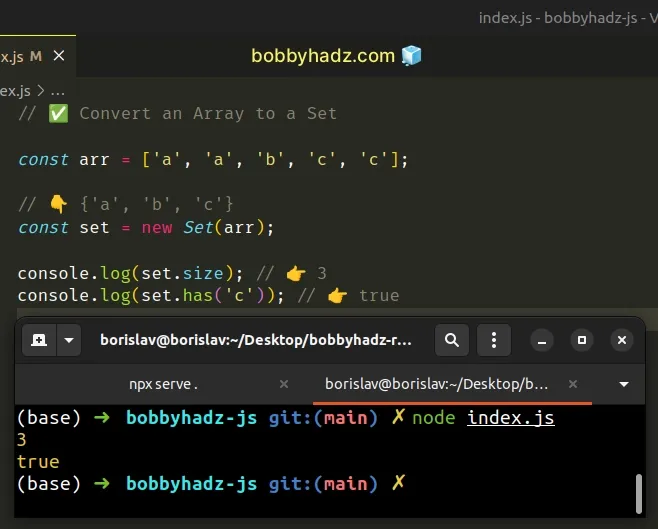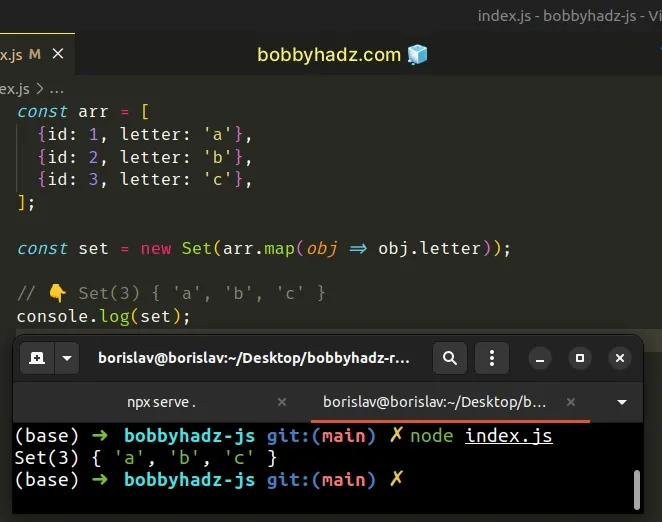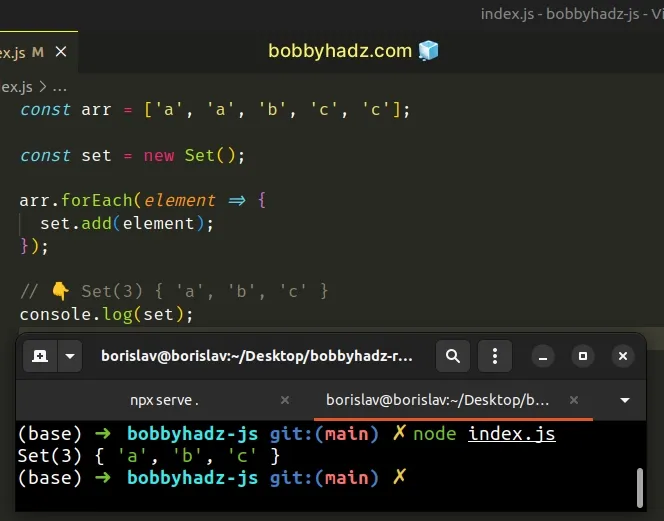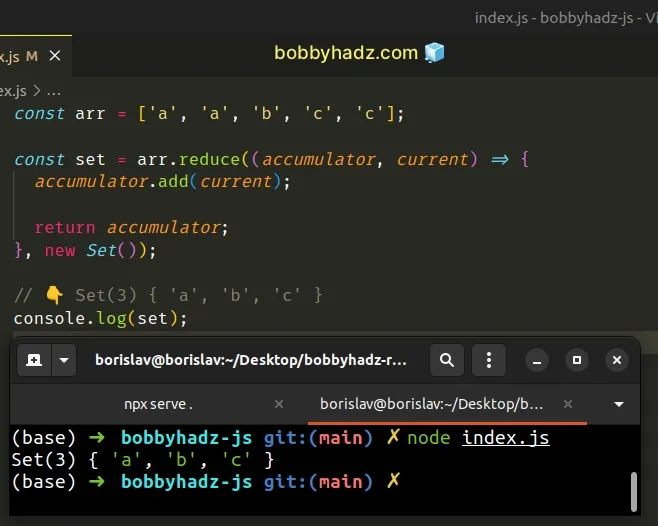# How to Convert an Array to a Set in JavaScriptLast updated: Dec 29, 2022
5 min## #Convert an Array to Set in JavaScript

Use the `Set()` constructor to convert an array to a `Set`, e.g. `const set = new Set(arr);`.

The `Set()` constructor is used to create `Set` objects that store unique values of any type.

index.js
```Copied!```// ✅ Convert an Array to a Set

const arr = ['a', 'a', 'b', 'c', 'c'];

// 👇️ {'a', 'b', 'c'}
const set = new Set(arr);

console.log(set.size); // 👉️ 3
console.log(set.has('c')); // 👉️ true

// ------------------------------------------

// ✅ Convert an Array of Objects to a Set

const arr2 = [
{id: 1, letter: 'a'},
{id: 2, letter: 'b'},
{id: 3, letter: 'c'},
];

const set2 = new Set(arr2.map(obj => obj.letter));

// 👇️ Set(3) { 'a', 'b', 'c' }
console.log(set2);
``````The Set object only stores unique values. Even if the supplied array contains duplicates, they don't get added to the `Set`.

index.js
```Copied!```const arr = ['a', 'a', 'a'];

const set = new Set(arr);

console.log(set); // 👉️ Set(1) { 'a' }

console.log(set.size); // 👉️ 1
``````

The only parameter the Set() constructor takes is an iterable.

When you pass an array to the `Set()` constructor, all of the array's elements get added to the `Set` (without the duplicates).

If you need to convert the `Set` back to an array, use the spread syntax (...).

index.js
```Copied!```const arr = ['a', 'a', 'b', 'c', 'c'];

// 👇️ {'a', 'b', 'c'}
const set = new Set(arr);

const newArr = [...set];

// 👇️ ['a', 'b', 'c']
console.log(newArr);
``````

The spread syntax (...) unpacks the values of the `Set` into the new array.

You can also use the `Array.from()` method to convert the `Set` back to an array.

index.js
```Copied!```const arr = ['a', 'a', 'b', 'c', 'c'];

// 👇️ {'a', 'b', 'c'}
const set = new Set(arr);

const newArr = Array.from(set);

// 👇️ ['a', 'b', 'c']
console.log(newArr);
``````

The `Array.from()` method creates an array from the provided iterable.

Note that all the duplicate values the old array had won't be contained in the new array.

If you need to convert an array of objects to a `Set` containing only the values of a specific property, use the `Array.map()` method.

## #Convert an Array of Objects to a Set

To convert an Array of objects to a `Set`:

1. Use the `Array.map()` method to iterate over the array.
2. On each iteration, access a specific property and return its value.
3. Use the `Set()` constructor to convert the array to a `Set` object.
index.js
```Copied!```const arr = [
{id: 1, letter: 'a'},
{id: 2, letter: 'b'},
{id: 3, letter: 'c'},
];

const set = new Set(arr.map(obj => obj.letter));

// 👇️ Set(3) { 'a', 'b', 'c' }
console.log(set);
``````The function we passed to the Array.map() method gets called with each element (object) in the array.

On each iteration, we access the `letter` property on the object and return the result.

index.js
```Copied!```const arr = [
{id: 1, letter: 'a'},
{id: 2, letter: 'b'},
{id: 3, letter: 'c'},
];

// 👇️ ['a', 'b', 'c']
console.log(arr.map(obj => obj.letter));

const set = new Set(arr.map(obj => obj.letter));

// 👇️ Set(3) { 'a', 'b', 'c' }
console.log(set);
``````

The output of the `Array.map()` method is an array containing all of the values of the `letter` property.

The last step is to pass the array of values to the `Set()` constructor.

## #Convert an Array to a Set using Array.forEach()

This is a three-step process:

1. Declare a new variable that stores an empty `Set` object.
2. Use the `Array.forEach()` method to iterate over the array.
3. On each iteration, use the `Set.add()` method to add the element to the `Set`.
index.js
```Copied!```const arr = ['a', 'a', 'b', 'c', 'c'];

const set = new Set();

arr.forEach(element => {
});

// 👇️ Set(3) { 'a', 'b', 'c' }
console.log(set);
``````The function we passed to the Array.forEach method gets called with each element in the array.

On each iteration, we use the `set.add()` method to add the element to a new `Set` object.

The Set.add method inserts a new element with the supplied value into a `Set` object, if the value is not already in the `Set`.

This approach is a bit more verbose than passing the array directly to the `Set` constructor, but it is useful when you need to process the values before adding them to the `Set`.

You can also use the `Array.reduce()` method to convert an array to a `Set` object.

## #Convert an Array to a Set using Array.reduce()

This is a three-step process:

1. Use the `Array.reduce()` method to iterate over the array.
2. Initialize the `accumulator` variable to an empty `Set`.
3. On each iteration, add the element to the `Set`.
index.js
```Copied!```const arr = ['a', 'a', 'b', 'c', 'c'];

const set = arr.reduce((accumulator, current) => {

return accumulator;
}, new Set());

// 👇️ Set(3) { 'a', 'b', 'c' }
console.log(set);
``````The function we passed to the Array.reduce method gets called for each element in the array.

The initial value of the `accumulator` variable is an empty `Set` because that's what we passed as the second argument to the `Array.reduce()` method.

On each iteration, we use the `Set.add()` method to add the current element to the `Set` object.

## #Add Array of Values to an Existing Set using spread syntax (...)

This is a three-step process:

1. Create a new `Set` using the `Set()` constructor.
2. Unpack the values of the existing `Set` and the array into a new `Set`.
3. The new `Set` will contain the values of the original `Set` and the array.
index.js
```Copied!```const set1 = new Set();
const arr = ['bobby', 'hadz', 'com'];

const newSet = new Set([...set1, ...arr]);
console.log(newSet); // 👉️ Set(3) { 'bobby', 'hadz', 'com' }
``````

We created a new `Set` using the `Set()` constructor and used the spread syntax (...) to unpack the values of the existing set and the array into the new `Set`.

Set objects and arrays are iterable, so we are able to use the spread syntax (...) to unpack them into a new `Set`.

You can also use a `for...of` loop to add an array of values to an existing `Set`.

## #Add an Array of Values to an Existing Set using a `for...of` loop

This is a two-step process:

1. Use a `for...of` loop to iterate over the array.
2. Use the `Set.add()` method to add each array element to the `Set` object.
index.js
```Copied!```const set1 = new Set();
const arr = ['bobby', 'hadz', 'com'];

for (const element of arr) {
}

console.log(set1); // 👉️ Set(3) { 'bobby', 'hadz', 'com' }
``````

The for...of statement is used to loop over iterable objects like arrays, strings, `Map`, `Set` and `NodeList` objects and `generators`.

On each iteration, we use the `Set.add()` method to add the current element to the `Set` object.